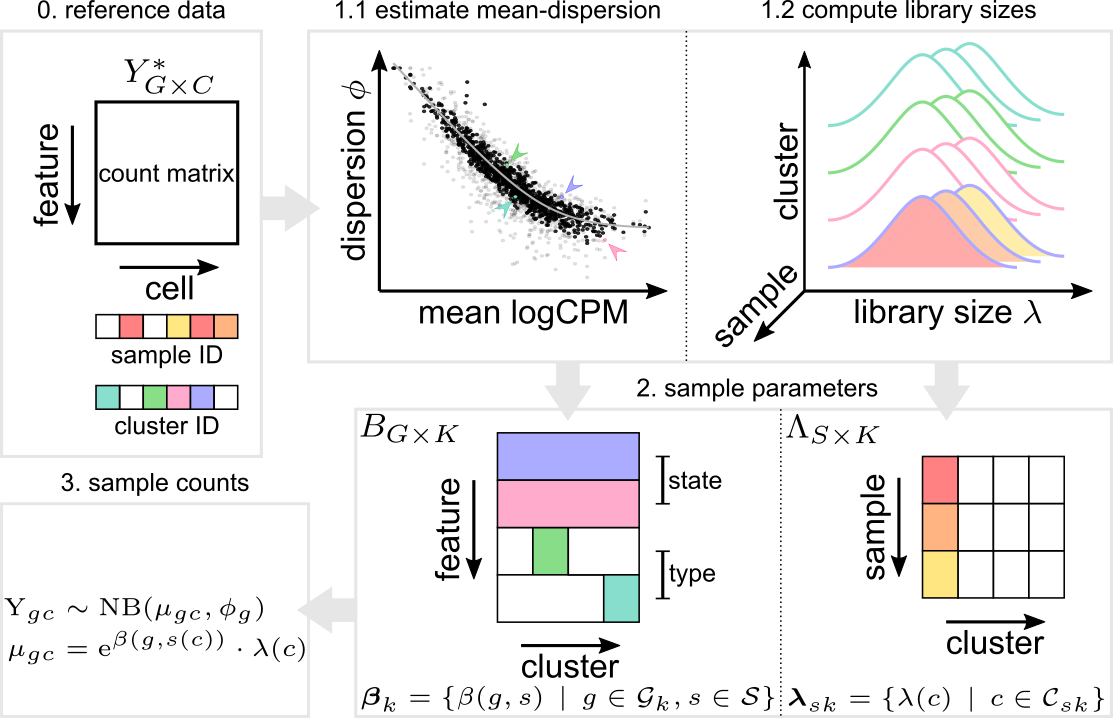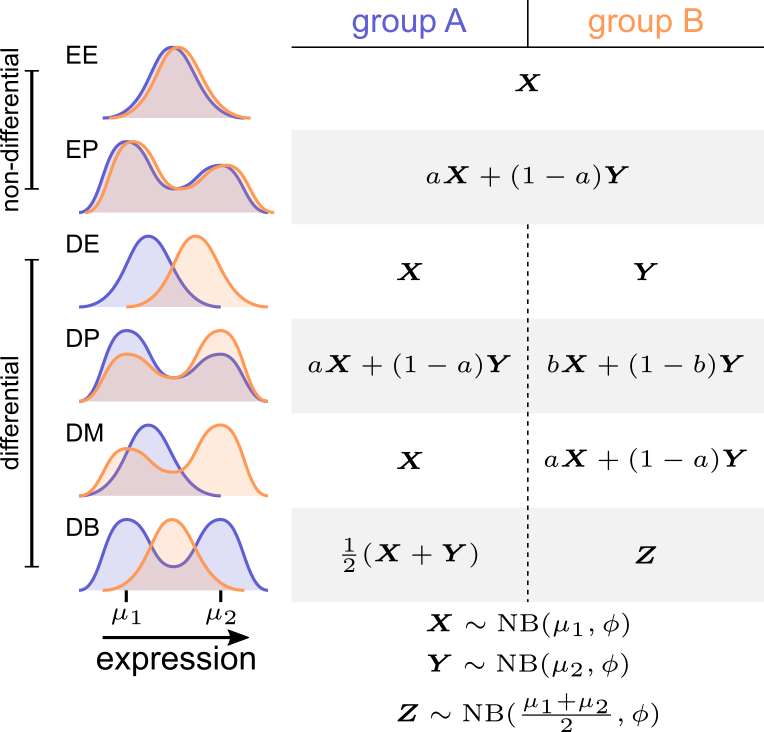# Contents

For details on the concepts presented here, consider having a look at our preprint:

Crowell HL, Soneson C*, Germain P-L*,
Calini D, Collin L, Raposo C, Malhotra D & Robinson MD:
On the discovery of population-specific state transitions from
multi-sample multi-condition single-cell RNA sequencing data.
bioRxiv 713412 (July, 2019). doi: 10.1101/713412

library(cowplot)
library(dplyr)
library(reshape2)
library(muscat)
library(purrr)
library(scater)
library(SingleCellExperiment)

# Data description

To demonstrate muscat’s simulation framework, we will use a SingleCellExperiment (SCE) containing 10x droplet-based scRNA-seq PBCM data from 8 Lupus patients obtained befor and after 6h-treatment with IFN-$$\beta$$ (Kang et al. 2018). The complete raw data, as well as gene and cell metadata is available through the NCBI GEO, accession number GSE96583.

# 1 Simulation framework

muscat’s simulation framework comprises: i) estimation of negative binomial (NB) parameters from a reference multi-subpopulation, multi-sample dataset; ii) sampling of gene and cell parameters to use for simulation; and, iii) simulation of gene expression data as NB distributions of mixtures thereof. See Fig. 1.

Let $$Y = (y_{gc})\in\mathbb{N}_0^{G\times C}$$ denote the count matrix of a multi-sample multi-subpopulation reference dataset with genes $$\mathcal{G} = \{ g_1, \ldots, g_G \}$$ and sets of cells $$\mathcal{C}_{sk} = \{ c^{sk}_1, ..., c^{sk}_{C_{sk}} \}$$ for each sample $$s$$ and subpopulation $$k$$ ($$C_{sk}$$ is the number of cells for sample $$s$$, subpopulation $$k$$). For each gene $$g$$, we fit a model to estimate sample-specific means $$\beta_g^s$$, for each sample $$s$$, and dispersion parameters $$\phi_g$$ using ’s function with default parameters. Thus, we model the reference count data as NB distributed:

$Y_{gc} \sim NB(\mu_{gc}, \phi_g)$

for gene $$g$$ and cell $$c$$, where the mean $$\mu_{gc} = \exp(\beta_{g}^{s(c)}) \cdot \lambda_c$$. Here, $$\beta_{g}^{s(c)}$$ is the relative abundance of gene $$g$$ in sample $$s(c)$$, $$\lambda_c$$ is the library size (total number of counts), and $$\phi_g$$ is the dispersion.Figure 1: Schematic overview of muscat’s simulation framework
Given a count matrix of features by cells and, for each cell, pre-determined subpopulation identifiers as well as sample labels (0), dispersion and sample-wise means are estimated from a negative binomial distribution for each gene (for each subpopulation) (1.1); and library sizes are recorded (1.2). From this set of parameters (dispersions, means, library sizes), gene expression is sampled from a negative binomial distribution. Here, genes are selected to be “type” (subpopulation-specifically expressed; e.g., via marker genes), “state” (change in expression in a condition-specific manner) or equally expressed (relatively) across all samples (2). The result is a matrix of synthetic gene expression data (3).

For each subpopulation, we randomly assign each gene to a given differential distribution (DD) category (Korthauer et al. 2016) according to a probability vector p_dd $$=(p_{EE},p_{EP},p_{DE},p_{DP},p_{DM},p_{DB})$$. For each gene and subpopulation, we draw a vector of fold changes (FCs) from a Gamma distribution with shape parameter $$\alpha=4$$ and rate $$\beta=4/\mu_\text{logFC}$$, where $$\mu_\text{logFC}$$ is the desired average logFC across all genes and subpopulations specified via argument lfc. The direction of differential expression is randomized for each gene, with equal probability of up- and down-regulation.

Next, we split the cells in a given subpopulations into two sets (representing treatment groups), $$\mathcal{T}_A$$ and $$\mathcal{T}_B$$, which are in turn split again into two sets each (representing subpopulations within the given treatment group.), $$\mathcal{T}_{A_1}/\mathcal{T}_{A_2}$$ and $$\mathcal{T}_{B_1}/\mathcal{T}_{B_2}$$.

For EE genes, counts for $$\mathcal{T}_A$$ and $$\mathcal{T}_B$$ are drawn using identical means.For EP genes, we multiply the effective means for identical fractions of cells per group by the sample FCs, i.e., cells are split such that $$\dim\mathcal{T}_{A_1} = \dim\mathcal{T}_{B_1}$$ and $$\dim\mathcal{T}_{A_2} = \dim\mathcal{T}_{B_2}$$. For DE genes, the means of one group, $$A$$ or $$B$$, are multiplied with the samples FCs. DP genes are simulated analogously to EP genes with $$\dim\mathcal{T}_{A_1} = a\cdot\dim\mathcal{T}_A$$ and $$\dim\mathcal{T}_{B_1} = b\cdot\dim\mathcal{T}_B$$, where $$a+b=1$$ and $$a\neq b$$. For DM genes, 50% of cells from one group are simulated at $$\mu\cdot\text{logFC}$$. For DB genes, all cells from one group are simulated at $$\mu\cdot\text{logFC}/2$$, and the second group is split into equal proportions of cells simulated at $$\mu$$ and $$\mu\cdot\text{logFC}$$, respectively. See Fig. 2.Figure 2: Schematic of the various types of differential distributions supported by muscat’s simulation framework
Differential distributions are simulated from a NB distribution or mixtures thereof, according to the definitions of random variables X, Y and Z.

## 1.1prepSim: Preparing data for simulation

To prepare a reference SingleCellExperiment (SCE) for simulation of multi-sample multi-group scRNA-seq data, prepSim will

1. perform basic filtering of genes and cells
2. (optionally) filter for subpopulation-sample instances with a threshold number of cells to assure accurate parameter estimation
3. estimate cell (library sizes) and gene parameters (dispersions and sample-specific means)

Importantly, we want to introduce known changes in states across conditions; thus, only replicates from a single condition should go into the simulation. The group to be kept for simulation may be specified via group_keep, in which case samples from all other groups (sce$group_id != group_keep) will be dropped. By default (group_keep = NULL), prepSim will select the first group available as reference. Arguments min_count, min_cells, min_genes and min_size are used to tune the filtering of genes, cells and subpopulation-instances as follows: • only genes with a count > min_count in >= min_cells will be retained • only cells with a count > 0 in >= min_genes will be retained • only subpopulation-sample instances with >= min_size cells will be retained; min_size = NULL will skip this step # estimate simulation parameters data(example_sce) ref <- prepSim(example_sce, verbose = FALSE) # only samples from ctrl group are retained table(ref$sample_id)
##
## ctrl101 ctrl107
##     200     200
# cell parameters: library sizes
sub <- assay(example_sce[rownames(ref), colnames(ref)])
all.equal(exp(ref$offset), as.numeric(colSums(sub))) ##  "names for target but not for current" ##  "Mean relative difference: 0.4099568" # gene parameters: dispersions & sample-specific means head(rowData(ref)) ## DataFrame with 6 rows and 4 columns ## ENSEMBL SYMBOL beta disp ## <character> <character> <DataFrame> <numeric> ## ISG15 ENSG00000187608 ISG15 -7.84574:-0.24711310:-1.039480 4.6360532 ## AURKAIP1 ENSG00000175756 AURKAIP1 -7.84859: 0.00768812:-1.171896 0.1784345 ## MRPL20 ENSG00000242485 MRPL20 -8.31434:-0.58684477:-0.304827 0.6435324 ## SSU72 ENSG00000160075 SSU72 -8.05160:-0.17119703:-0.793222 0.0363892 ## RER1 ENSG00000157916 RER1 -7.75327: 0.10731331:-1.261821 0.5046166 ## RPL22 ENSG00000116251 RPL22 -8.03553:-0.03357193: 0.143506 0.2023632 ## 1.2simData: Simulating complex designs Provided with a reference SCE as returned by prepSim, a variery of simulation scenarios can be generated using the simData function, which will again return an SCE containg the following elements: • assay counts containing the simulated count data • colData columns cluster/sample/group_id containing each cells cluster, sample, and group ID (A or B). • metadata$gene_info containing a data.frame listing, for each gene and cluster
• the simulationed DD category
• the sampled logFC; note that this will only approximate log2(sim_mean.B/sim_mean.A) for genes of the de category as other types of state changes use mixtures for NBs, and will consequently not exhibit a shift in means of the same magnitude as logFC
• the reference sim_gene from which dispersion sim_disp and sample-specific means beta.<sample_id> were used
• the simulated expression means sim_mean.A/B for each group

In the code chunk that follows, we run a simple simulation with

• p_dd = c(1,0,...0), i.e., 10% of EE genes
• nk = 3 subpopulations and ns = 3 replicates for each of 2 groups
• ng = 1000 genes and nc = 2000 cells, resulting in 2000/2/ns/nk $$\approx111$$ cells for 2 groups with 3 samples each and 3 subpopulations
# simulated 10% EE genes
sim <- simData(ref, p_dd = diag(6)[1, ],
nk = 3, ns = 3, nc = 2e3,
ng = 1e3, force = TRUE)
# number of cells per sample and subpopulation
table(sim$sample_id, sim$cluster_id)
##
##             cluster1 cluster2 cluster3
##   sample1.A      108      144      117
##   sample2.A      128      105      101
##   sample3.A      120       94      123
##   sample1.B      110      103      103
##   sample2.B       98      105      118
##   sample3.B      111      112      100

By default, we have drawn a random reference sample from levels(ref$sample_id) for every simulated sample in each group, resulting in an unpaired design: metadata(sim)$ref_sids
##         A         B
## sample1 "ctrl107" "ctrl101"
## sample2 "ctrl107" "ctrl101"
## sample3 "ctrl101" "ctrl107"

Alternatively, we can re-run the above simulation with paired = TRUE such that both groups will use the same set of reference samples, resulting in a paired design:

# simulated paired design
sim <- simData(ref, paired = TRUE,
nk = 3, ns = 3, nc = 2e3,
ng = 1e3, force = TRUE)
# same set of reference samples for both groups
ref_sids <- metadata(sim)$ref_sids all(ref_sids[, 1] == ref_sids[, 2]) ##  TRUE ### 1.2.1p_dd: Simulating differential distributions Argument p_dd specifies the fraction of cells to simulate for each DD category. Its values should thus lie in $$[0,1]$$ and sum to 1. Expression densities for an exemplary set of genes simulated from the code below is shown in Fig. 3. # simulare genes from all DD categories sim <- simData(ref, p_dd = c(0.5, rep(0.1, 5)), nc = 2e3, ng = 1e3, force = TRUE) We can retrieve the category assigned to each gene in each cluster from the gene_info table stored in the output SCE’s metadata: gi <- metadata(sim)$gene_info
table(gi\$category)
##
##  ee  ep  de  dp  dm  db
## 980 194 234 194 188 210